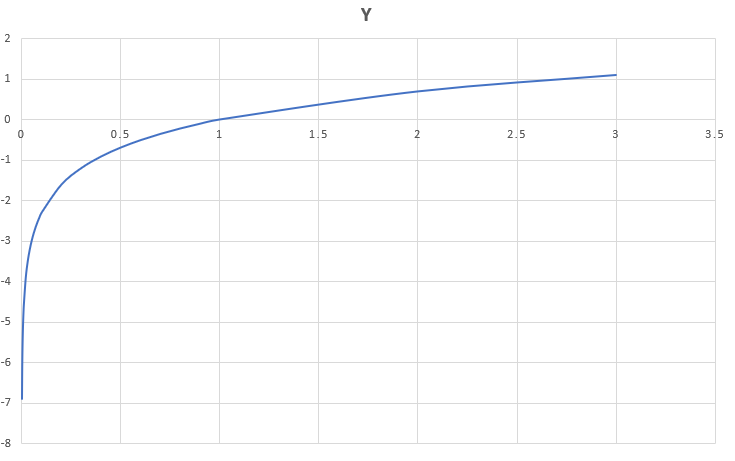# log() function in C++

Log() function in C++ : The log() function in C++ returns the natural logarithm (base-e logarithm) of the argument passed in the parameter.Syntax for returning natural logarithm:
result = log(x)

Syntax for returning logarithm (base-10 logarithm) of the argument.
result = log10(x)

The parameters can be of any data-type like int, double or float or long double.

Log() function returns value according to the following conditions:

..a) if x>1 then positive
..b) if 0<x<1 returns a negative value
..c) if x=1 then it returns 0
..d) if x=0 then it returns -inf
..e) if x<0 then it returns NaN(not a number)

 `// CPP program to implement log() function ` `#include ` `using` `namespace` `std; ` ` `  `// function to evaluate natural logarithm base-e ` `double` `valueE(``double` `d) ` `{ ` `    ``return` `log``(d); ` `} ` ` `  `// function to evaluate logarithm base-10 ` `double` `value10(``double` `d) ` `{ ` `    ``return` `log10``(d); ` `} ` ` `  `// driver program to test the above function ` `int` `main() ` `{ ` `    ``double` `d = 10; ` `    ``cout << ``"The logarithm value(base-e) of "` `<< d  ` `         ``<< ``" is "` `<< valueE(d) << endl; ` `    ``cout << ``"The logarithm value(base-10) of "` `<< d  ` `         ``<< ``" is "` `<< value10(d) << endl; ` `    ``return` `0; ` `} `

Output:

```The logarithm value(base-e) of 10 is 2.30259
The logarithm value(base-10) of 10 is 1
```

Application:
One of the applications of log() function is to calculated values related to log, for e.g., while finding polite number we need the formula to be written in code, for that we can use log() function. Given below is an implementation of log() function.

 `// CPP program to find Nth polite number ` `#include ` `using` `namespace` `std; ` ` `  `// function to evaluate n-th polite number ` `double` `polite(``double` `n) ` `{ ` `    ``n += 1; ` `    ``double` `base = 2; ` `    ``return` `n + (``log``((n + (``log``(n) / ` `                 ``log``(base))))) / ``log``(base); ` `} ` ` `  `// driver code ` `int` `main() ` `{ ` `    ``double` `n = 7; ` `    ``cout << (``int``)polite(n); ` `    ``return` `0; ` `} `

Output:

```11
```

Attention reader! Don’t stop learning now. Get hold of all the important DSA concepts with the DSA Self Paced Course at a student-friendly price and become industry ready.

My Personal Notes arrow_drop_upCheck out this Author's contributed articles.

If you like GeeksforGeeks and would like to contribute, you can also write an article using contribute.geeksforgeeks.org or mail your article to contribute@geeksforgeeks.org. See your article appearing on the GeeksforGeeks main page and help other Geeks.

Please Improve this article if you find anything incorrect by clicking on the "Improve Article" button below.

Improved By : mathemagic, a7medsaber

Article Tags :
Practice Tags :

4

Please write to us at contribute@geeksforgeeks.org to report any issue with the above content.